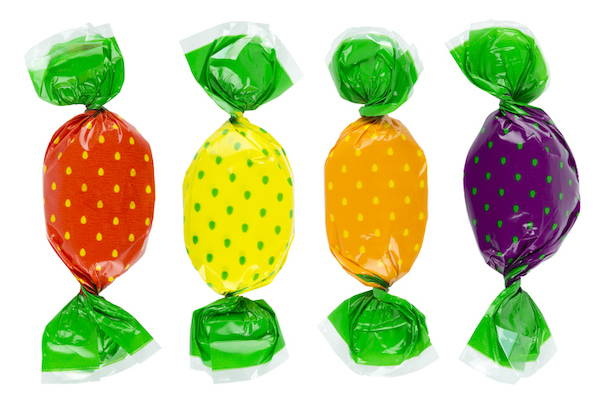# Ratio and Proportion

## What is ratio?

Ratio compares one amount to another amount.

A ratio is written using a colon : between the amounts.

e.g. For every three sweets that Aaron eats, Julie eats one sweet.

This would be written as a ratio as 3:1

This means that for every group of four sweets, Aaron eats three of them, and Julie eats one of them.## What is proportion?

Proportion tells us about a part of an amount in relation to the whole amount.

e.g. There are four sweets. Aaron eats three out of the four sweets. Julie eats one out of the four sweets.

Proportions can be expressed as fractions. In this example, Aaron eats 3/4 of the sweets, and Julie eats 1/4 of them.

## Ratio questions

1. In my garden, there are 8 blackbirds for every 6 sparrows. How could we write this as a ratio in its simplest form?

The ratio can be written as 8:6

In order to simplify this, we need to divide both amounts by the same number.

We can divide both of the numbers in the ratio by 2:

8 ÷ 2 = 4

6 ÷ 2 = 3

So, the ratio in its simplest form can be written as 4:3.

(Sometimes you might be able to divide the amounts again by another number. You will know when you have reached the simplest form when you cannot divide both amounts by the same number any further.)

2. There are 24 ladybirds on a leaf. The ratio of red to yellow ladybirds is 10:2. How many red ladybirds are there?

We could solve this problem using a diagram, where 'L' stands for one ladybird.

After every 10 'L's to represent the red laybirds, there should be 2 'L's to represent the yellow ladybirds. Continue drawing 'L's until you have 24.

When counted, we can see that there are 20 red ladybirds on the leaf.

Here is another way of solving the above problem:

Add the numbers in the ratio (10:2) together:

10 + 2 = 12

Divide the total number of ladybirds (24) by this number (12):

24 ÷ 12 = 2

To find out how many red ladybirds there are, multiply 10 by 2:

10 x 2 = 20.

There are 20 red ladybirds on the leaf.

## Proportion questions

1. There are 21 coins in a jar. Two in every seven coins are silver. How many silver coins are there in the jar?

Again, we could use a diagram to solve this problem:

🟡 🟡 🟡 🟡 🟡 ⚪️ ⚪️

🟡 🟡 🟡 🟡 🟡 ⚪️ ⚪️

🟡 🟡 🟡 🟡 🟡 ⚪️ ⚪️

When counted, we can see that there are 6 silver coins in the jar.

Here is another way of solving the problem:

First, calculate how many groups of seven coins there are in the jar:

21 ÷ 7 = 3

Now, multiply the number of silver coins in every group of seven by 3:

2 x 3 = 6

There are 6 silver coins in the jar.

2. In a box of chocolates, 3 in every 8 are toffee-flavoured. If there are 48 chocolates in the box althogether, how many are toffee-flavoured?

It would take a long time to draw a diagram of 48 chocolates, so for this question, using division and multiplication skills are more efficient.

First, calculate how many groups of eight chocolates there are in the box:

48 ÷ 8 = 6

Now, multiply the number of toffee-flavoured chocolates in every group of eight by 6:

3 x 6 = 18

There are 18 toffee-flavoured chocolates in the box.

## Ratio and Proportion Year 6

Ratio and Proportion appears in the National Curriculum in Year 6.

Within this strand, pupils are taught to:

- solve problems involving the relative sizes of two quantities where missing values can be found by using integer multiplication and division facts 

- solve problems involving the calculation of percentages [for example, of measures, and such as 15% of 360] and the use of percentages for comparison 

- solve problems involving similar shapes where the scale factor is known or can be found 

- solve problems involving unequal sharing and grouping using knowledge of fractions and multiples.